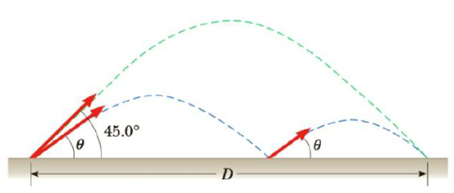Chapter 3, Problem 48AP

Chapter
Section
Textbook Problem

When baseball outfielders throw the ball, they usually allow it to take one bounce, on the theory that the ball arrives at its target sooner that way. Suppose that, after the bounce, the ball rebounds at the same angle θ that it had when it was released (as in Fig. P3.48), but loses half its speed, (a) Assuming that the ball is always thrown with the same initial speed, at what angle θ should the ball be thrown in order to go the same distance D with one bounce as a ball thrown upward at 45.0° with no bounce? (b) Determine the ratio of the times for the one-bounce and no-bounce throws.Figure P3.48

(a)

To determine
The angle with which the ball be thrown in order to go the same distance as with one bounce as a ball thrown at 45.0° with no bounce.

Explanation

When the angle is 45.0° , the time of flight is,

Δy=v0yt+12ayt2

Here,

y is the vertical displacement

y0 is the initial position in the vertical direction

v0y is the vertical component of the initial velocity

ay is the vertical acceleration

t is the time taken

0=(v02)t1g2t12t1=v02g

The horizontal range of this ball is,

R1=v0xt1

Here,

v0x is the horizontal component of the initial velocity

R1=(v02)(v02g)=v02g

Considering the first arc of the second ball,

Δy=v0yt+12ayt20=(v0sinθ)t21g2t212t21=2v0sinθg

For the second arc,

t22=2(v02)

(b)

To determine
The ratio of the times for the one-bounce and no-bounce throws.

Still sussing out bartleby?

Check out a sample textbook solution.

See a sample solution

The Solution to Your Study Problems

Bartleby provides explanations to thousands of textbook problems written by our experts, many with advanced degrees!

Get Started